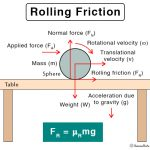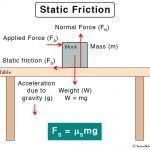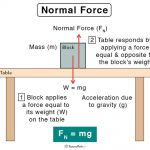Home / Physics / Archimedes’ Principle

# Archimedes’ Principle

## Definition: What is Archimedes’ Principle?

Archimedes’ principle is a law of physics fundamental to fluid dynamics. It states that the upward buoyant force exerted on a body immersed in a fluid, whether wholly or partially submerged, is equal to the weight of the fluid that the body displaces. If the weight of the fluid displaced is less than the weight of the object, the object will sink. The object will float if the weight of the fluid displaced is equal to the weight of the object. This upward force is known as thrust or buoyant force. It is a consequence of the difference in pressure the fluid exerts at different heights. The fluid pressure is exerted in all directions (Pascal’s principle) and increases with depth. There is an unbalanced upward force on the bottom of a submerged object.

### History of Archimedes’ Principle

The history of this principle goes back to 3rd century BC when it was discovered by Archimedes of Syracuse, who was a Greek mathematician, physicist, engineer, inventor, and astronomer. This discovery is often associated with the term “Eureka moment.”

According to the popular legend, Archimedes was given the job of finding out whether a crown made for a king was either solid gold or fake gold. One day he was taking a bath and noticed how the water level rose as he immersed his body. Realizing that if a gold crown were dropped into a bath, it would displace its volume of water over the side. This idea, effectively, gave him an easy way to measure the crown’s volume. By weighing the crown, he could then efficiently work out its density (mass divided by the volume) and compare it with that of gold. If the density was lower than that of gold, the crown was a fake.

## Examples of Archimedes’ Principle

Archimedes principle is a commonly occurring phenomenon in real life. Some notable examples are as follows.

Image Courtesy: Satyam Bhuyan

• A ship or a boat can float on water because the weight of the displaced water is equal to the weight of the ship or boat.
• A submarine can dive into the water or can float on it. A submarine consists of ballast tanks that are filled with air or water. The tanks weigh less than an equal volume of water and make the sub float on the surface. If the tanks are partly filled with air, it is possible to make the submarine float at some depth of the water without either rising or sinking.
• Hot air balloons rise into the air because the density of the warmer air inside the balloon is less dense than the colder air outside. The basic principle of hot air balloon is the use of hot air to create buoyancy, which generates lift.
• Water striders can float on the water since its legs expel water, and the weight of the expelled water is equal to the floating force.

## Theory of Archimedes’ Principle

The thrust provided by the fluid opposes the weight due to gravity. The object inside the fluid only feels the total force acting on it, which is called the apparent weight. Because the fluid’s thrust decreases the actual gravitational force, the object feels as though its weight is reduced. The apparent weight is given by,

Apparent weight = Weight of the object in the air – Thrust or buoyant force

## Archimedes’ Principle Equation

Archimedes’ principle tells us that this loss of weight is equal to the weight of the fluid, wholly or partially, displaced by the object. The corresponding equation is given by,

Fb = ρ X g X V

Where,

Fb is the buoyant force (or thrust)

ρ is the density of the fluid in which the object is immersed

V is the volume of the object that is submerged in the fluid

g is the acceleration due to gravity

## Derivation Archimedes’ Principle Formula: Law of Buoyancy

The mass of the liquid displaced is,

Mass = Density X Volume

M = ρ X V

The weight of the displaced liquid is,

Weight = Mass X Gravity

W = M X g

Or, W = ρ X V X g

According to Archimedes’ principle,

Apparent loss of weight = Weight of the displaced liquid

This apparent loss of weight is the thrust or buoyant force (Fb). Therefore,

Fb = ρ X g X V

It is this force that is responsible for objects to float. Thus, this equation is also called the law of buoyancy.

## Uses and Applications of Archimedes’ Principle

Archimedes’ principle is applied to the following situation.

• An essential application of the Archimedes’ principle is to measure the volume and density of irregularly shaped objects.
• In a hydrometer, a solid is suspended in a fluid and buoyed by force equal to the weight of the fluid displaced by the submerged part.
• It is used in a large variety of scientific research subjects, including medical, engineering, entomology, engineering, and geology. For example, it is used to determine the densities of bones and teeth.

Article was last reviewed on Tuesday, July 7, 2020

### Related articlesRolling FrictionSliding FrictionStatic FrictionNormal Force